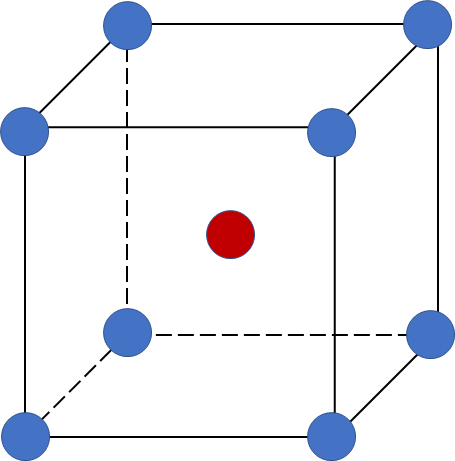# Problem: The density of solid Cr is 7.15 g/cm3. How many atoms are present per cubic centimeter of Cr? in atoms/cm^3As a solid, Cr adopts a body-centered cubic unit cell. How many unit cells are present per cubic centimeter of Cr? in unit cells/cm^3What is the volume of a unit cell of this metal? in cm^3What is the edge length of a unit cell of Cr? in cm

🤓 Based on our data, we think this question is relevant for Professor Walker's class at COLLIN.

###### FREE Expert Solution

a) number of atoms present per cubic centimeter of Cr (atoms/cm3)

b) number of unit cells present per cubic centimeter of Cr (unit cell/cm3)

c) volume of a unit cell of this metal (in cm3)

d) edge length of a unit cell of Cr (in cm)

Cr body-centered cubic (BCC) unit cell:

• cube with one atom at each of its corners and one atom at the center of the cube###### Problem Details

The density of solid Cr is 7.15 g/cm3. How many atoms are present per cubic centimeter of Cr? in atoms/cm^3

As a solid, Cr adopts a body-centered cubic unit cell. How many unit cells are present per cubic centimeter of Cr? in unit cells/cm^3

What is the volume of a unit cell of this metal? in cm^3

What is the edge length of a unit cell of Cr? in cm